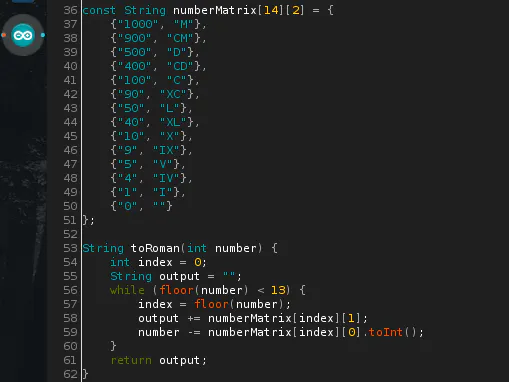Project tutorial# Roman Numeral Converter for Arduino © GPL3+

An easy software solution that can be implemented into bigger projects.

• 1,772 views
• 9 respects

## Apps and online services

I was considering recently to implement a dynamic part for my ESP8266 web server password. Roman numerals seemed like a nice idea since I only need to know the current time for that part of the password. After some research into this topic, I have not found any example online that worked for me. I tried to find Java example for a converter, and there is a very nice, elegant solution for that. After trying to replicate its logic, I have made a working code that converts Roman numerals to Arabic numbers and back. I hope you find it helpful.

The input string is evaluated to verify if it has numeric value or not:

``````boolean isValidNumber(String str) {
if (str.length() == 0) return false;
for (byte i = 0; i < str.length(); i++)
{
if (!isDigit(str.charAt(i))) return false;
}
return true;
}
``````

Based on the value of the string, the appropriate function is called:

``````if (isValidNumber(input)) {
Serial.print(" converted to Roman numerals is: ");
Serial.println(toRoman(input.toInt()));
} else {
Serial.print(" converted to Arabic numbers is: ");
Serial.println(toArabic(input));
}
``````

Both functions are working on the same principle. The input string is compared with all values from multidimensional array.

``````const String numberMatrix = {
{"1000", "M"},
{"900", "CM"},
{"500", "D"},
{"400", "CD"},
{"100", "C"},
{"90", "XC"},
{"50", "L"},
{"40", "XL"},
{"10", "X"},
{"9", "IX"},
{"5", "V"},
{"4", "IV"},
{"1", "I"},
{"0", ""}
};
``````

Every found value is removed from the input string and the result is added to the output.

If not needed, either to Arabic or to Roman functions can be removed with their number matrix and floor functions since both parts are independent.

Java code that helped me write this code is attached to this project.

## Code

##### Roman numerals converterC/C++
```/*
Roman numerals converter

This sketch is converting Roman numerals to Arabic numbers and back.
It is made by following the logic of Java example I found online.

Created 15 August 2018
By Dusan Lesan
*/

String input = "1884";
//String input = "MDCCCLXXXIV";

void setup() {
Serial.begin(9600);
Serial.print(input);
if (isValidNumber(input)) {
Serial.print(" converted to Roman numerals is: ");
Serial.println(toRoman(input.toInt()));
} else {
Serial.print(" converted to Arabic numbers is: ");
Serial.println(toArabic(input));
}
}

void loop() {}

boolean isValidNumber(String str) {
if (str.length() == 0) return false;
for (byte i = 0; i < str.length(); i++)
{
if (!isDigit(str.charAt(i))) return false;
}
return true;
}

const String numberMatrix = {
{"1000", "M"},
{"900", "CM"},
{"500", "D"},
{"400", "CD"},
{"100", "C"},
{"90", "XC"},
{"50", "L"},
{"40", "XL"},
{"10", "X"},
{"9", "IX"},
{"5", "V"},
{"4", "IV"},
{"1", "I"},
{"0", ""}
};

String toRoman(int number) {
int index = 0;
String output = "";
while (floor(number) < 13) {
index = floor(number);
output += numberMatrix[index];
number -= numberMatrix[index].toInt();
}
return output;
}

int floor(int number) {
int index = 0;
for (index; index < 14; index++) {
if (numberMatrix[index].toInt() <= number) {
return index;
}
}
return index;
}

const String reversedNumberMatrix = {
{"900", "CM"},
{"1000", "M"},
{"400", "CD"},
{"500", "D"},
{"90", "XC"},
{"100", "C"},
{"40", "XL"},
{"50", "L"},
{"9", "IX"},
{"10", "X"},
{"4", "IV"},
{"5", "V"},
{"1", "I"},
{"0", ""}
};

int toArabic(String romanNumber) {
int index = 0;
int output = 0;
while (floor(romanNumber) < 13) {
index = floor(romanNumber);
output += reversedNumberMatrix[index].toInt();
romanNumber.remove(romanNumber.indexOf(reversedNumberMatrix[index]), reversedNumberMatrix[index].length());
}
return output;
}
int floor(String number) {
int index = 0;
for (index; index < 14; index++) {
if (number.indexOf(reversedNumberMatrix[index]) != -1) {
return index;
}
}
return index;
}
```
##### Java code exampleJava
This is a code that helped me with the logic of my code
```import java.util.TreeMap;

public class RomanNumeralsConverter {

private final static TreeMap<Integer, String> map = new TreeMap<Integer, String>();

static {
map.put(1000, "M");
map.put(900, "CM");
map.put(500, "D");
map.put(400, "CD");
map.put(100, "C");
map.put(90, "XC");
map.put(50, "L");
map.put(40, "XL");
map.put(10, "X");
map.put(9, "IX");
map.put(5, "V");
map.put(4, "IV");
map.put(1, "I");
}

public static void main(String[] args) {
System.out.println(toRoman(5884));
}

public final static String toRoman(int number) {

int l =  map.floorKey(number);
if ( number == l ) {
return map.get(number);
}
return map.get(l) + toRoman(number-l);
}
}
```

August 17, 2018

#### Members who respect this project

See similar projects
you might like

• 3,487 views
• 6 respects

• 34,217 views
• 9 respects

#### Telegram Bot with ESP8266

Project tutorial by Shebin Jose Jacob

• 38,834 views
• 75 respects

• 11,291 views
• 11 respects

#### Morse Code Communication Using Arduino

Project tutorial by Jalal_Mansoori

• 11,514 views